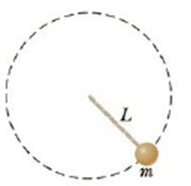Chapter 7, Problem 70AP

Chapter
Section
Textbook Problem

A 0.275-kg object is swung in a vertical circular path on a string 0.850 m long as in Figure P7.70. (a) What are the forces acting on the ball at any point along this path? (b) Draw free-body diagrams for the ball when it is at the bottom of the circle and when it is at the top. (c) If its speed is 5.20 m/s at the top of the circle, what is the tension in the string there? (d) If the string breaks when its tension exceeds 22.5 N, what is the maximum speed the object can have at the bottom before the string breaks?Figure P7.70

(a)

To determine
The forces on the ball.

Explanation

Given info: Mass of the ball (m) is 0.275 kg. Length of the string (L) is 0.850 m.

Explanation:

The force of gravity acts on the ball in the downward direction...

(b)

To determine
The free body diagram of the ball at the top and bottom.

(c)

To determine
The tension at the top of the circle.

(d)

To determine
The maximum speed of the ball at the bottom of the circle.

Still sussing out bartleby?

Check out a sample textbook solution.

See a sample solution

The Solution to Your Study Problems

Bartleby provides explanations to thousands of textbook problems written by our experts, many with advanced degrees!

Get Started

What is meant by the precision of a measurement? How is it indicated?

General Chemistry - Standalone book (MindTap Course List)

Which distance is farther, 100 km or 50 mi?

Introductory Chemistry: A Foundation

Define the following terms: a. chromosome b. chromatin

Human Heredity: Principles and Issues (MindTap Course List)